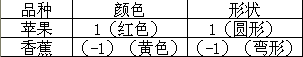# 深度学习之神经网络入门（一）

a1,a2,…,an是输入的信号，用w代表信号强度的权重。即有s=a1w1+a2w2+…+anwn。以上就是构造人工神经元的基本过程。即根据生物神经元的原理做的算法模拟，及接受刺激信号并汇总输出。但是，这样的话神经元还没有被激活，在信号输出端有一个传递函数f，通过这个传递函数来输出最终的结果。这个传递函数也叫激励函数，激励函数有以下几种：
1.阶梯函数：Step
2.符号函数：Sgn
3.线性函数：Linear
4.饱和线性函数：Ramp
5.对数S形函数：Sigmoid（常用的激励函数）
6.双曲正切S形函数：Tanh（1） 苹果的形状和颜色均输入属性1，有：s=1-1+0=0；f=0（step函数）

（2） 通过观察可知，期望结果为1即t=1，而实际得到0，即a=0；
e=t-a=1-0=1；
w1new=w1old+ep=1+1x1=2；w2new=w2old+ep=-1+1x1=0；bnew=bold+e=0+1=1；

（3）将新的权值和偏置带入感知机有：net=a1w1+a2w2+b=1x2+1x0+1=3，f（step）=1；

（4） 通过纠正误差后苹果判断正取，看一下香蕉的：
net=a1w1+a2w2+b=-1x2-1x0+1=-1，f（step）=0；